# 基于Keras构建卷积神经网络识别正方系统验证码

### 基于Keras构建卷积神经网络识别正方系统验证码http://211.83.32.12/CheckCode.aspx

### 下载验证码```def get_checkcode(i):
r = requests.get('http://211.83.32.12/CheckCode.aspx')  #这里填入验证码的url
picname = str(i) + '.png'
with open(picname, 'wb') as f:
f.write(r.content)```

### 二值化，去噪，切割验证码```def process_pic(i):
picname = str(i) + '.png'
im = Image.open(picname)
im = im.point(lambda i: <span style="color: #ff0000;">i == 3</span>, mode='1')
im_save =im.save(str(i) + '_after.png')
y_min, y_max = 0, 22
split_lines = [5, 17, 29, 41, 53]
ims = [im.crop([u, y_min, v, y_max]) for u, v in zip(split_lines[:-1], split_lines[1:])]
return ims```

im = im.point(lambda i: i == 3, mode=’1′)

point()方法通过一个函数或者查询表对图像中的像素点进行处理，其中lambda i遍历整张图片，3是一个阀值，等于3填充为1，不等于3填充为0，mode=’1’的意思是输出模式为整数型，由此实现二值化。

3这个值我试验了很久才找出来，细心观察上面验证码图片的同学会发现验证码中的字符的主体部分都是由深蓝色的点构成的，而这个深蓝色的像素点的值就是3。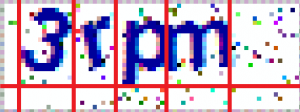y_min, y_max = 0, 22设定验证码图片中最大的y值和最小的y值。

zip(split_lines[:-1], split_lines[1:]) 运行结果为[(5,17),(17,29),(29,41),(41,53)]

im.crop([u, y_min, v, y_max]) ，crop()函数为用来复制一个图片里的一矩形内容，传入参数为矩形的四条边，这样保证切割处理的图片的长宽都是统一的。所以通过以上的crop()函数实现图片的分割。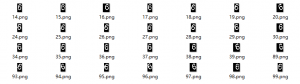```import tkinter as tk
from tkinter import messagebox
import requests
from PIL import Image, ImageTk
import os

def get_cnt():
try:
with open('count.txt', 'r') as f:
cnt = eval(cnt)
return cnt
except:
with open('count.txt', 'w') as f:
f.write('0')
return 0

def update_cnt(cnt):
with open('count.txt', 'w+') as f:
f.write(str(cnt))

def get_checkcode(i):
#随便找了个公网能访问的正方系统
r = requests.get('http://jwgl.uoh.edu.cn/CheckCode.aspx')
picname = str(i) + '.png'
with open(picname, 'wb') as f:
f.write(r.content)

def process_pic(i):
picname = str(i) + '.png'
im = Image.open(picname)
im = im.point(lambda i: i == 3, mode='1')
im_save =im.save(str(i) + '_after.png')
y_min, y_max = 0, 22
split_lines = [5, 17, 29, 41, 53]
ims = [im.crop([u, y_min, v, y_max]) for u, v in zip(split_lines[:-1], split_lines[1:])]
return ims

def get_pic_for_display(i):
last1 = str(i-1)+'.png'
last2 = str(i-1)+'_after.png'
if os.path.exists(last1):
os.remove(last1)
os.remove(last2)
picname = str(i) + '.png'
im = Image.open(picname)
w, h = im.size
w_box = 300
h_box = 200
im_resized = resize(w, h, w_box, h_box, im)
tk_image = ImageTk.PhotoImage(im_resized)
return tk_image

def display_after(i):
picname = str(i) + '_after.png'
im = Image.open(picname)
w, h = im.size
w_box = 300
h_box = 200
im_resized = resize(w, h, w_box, h_box, im)
tk_image = ImageTk.PhotoImage(im_resized)
return tk_image

def resize(w, h, w_box, h_box, pil_image):
f1 = w_box / w
f2 = h_box / h
factor = min([f1, f2])
width = int(w * factor)
height = int(h * factor)
return pil_image.resize((width, height), Image.ANTIALIAS)

def display_pic():
global im
tmp = get_cnt() + 1
get_checkcode(tmp)
im = tk.PhotoImage(file=str(tmp) + '.png')
im = get_pic_for_display(tmp)
picLabel['image'] = im
cntLabel['text'] = '第' + str(tmp - 1) + '张验证码'
global ims
ims = process_pic(tmp)
display_after_pic()

def display_after_pic():
global im2
tmp = get_cnt() + 1
get_checkcode(tmp)
im2 = tk.PhotoImage(file=str(tmp) + '_after.png')
im2 = display_after(tmp)
picLabel_after['image'] = im2

def save_imgs():
tmp = get_cnt() + 1
code = var.get()
for i in range(4):
BASE_DIR = os.path.dirname(os.path.realpath(__file__))
path = os.path.join(BASE_DIR, 'split-str', code[i])
if os.path.exists(path):
filepath = os.path.join(path, 'count.txt')
with open(filepath, 'r') as f:
else:
os.makedirs(path)
filepath = os.path.join(path, 'count.txt')
with open(filepath, 'w') as f:
f.write('0')
char_cnt = 0
charname = os.path.join(path, str(char_cnt + 1) + '.png')
ims[i].save(charname)
filepath = os.path.join(path, 'count.txt')
with open(filepath, 'w+') as f:
f.write(str(char_cnt + 1))
update_cnt(tmp)

def submit():
save_imgs()
display_pic()
var.set('')

def init():
messagebox.showinfo("初始化窗口", "标记前请确保已连接网络!\n标记后回车即可下一张！")
display_pic()

def sub_event(event):
if event.keysym == 'Return':
submit()

global im,im2
app = tk.Tk()
app.title('正方系统验证码标记工具  --Zgao')
app.geometry('500x360+400+220')
picLabel = tk.Label(app)
picLabel.pack()
picLabel_after = tk.Label(app)
picLabel_after.pack()
var = tk.StringVar()
textInput = tk.Entry(app, textvariable=var,width=10)
submitButton = tk.Button(app, text="确定", width='10', command=submit)
submitButton.bind_all('&lt;KeyPress&gt;', sub_event)
submitButton.pack()
cntLabel = tk.Label(app)
init()
app.mainloop()```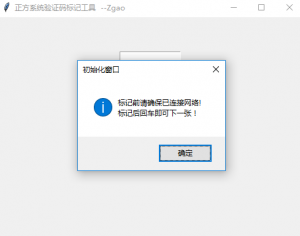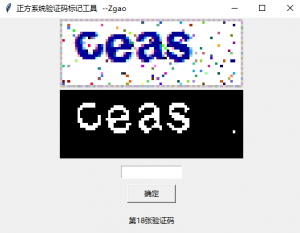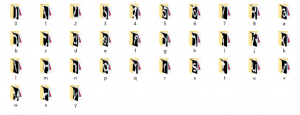##### Keras 中文文档

Keras 是一个用 Python 编写的高级神经网络 API，它能够以 TensorFlowCNTK, 或者 Theano 作为后端运行。Keras 的开发重点是支持快速的实验。能够以最小的时延把你的想法转换为实验结果，是做好研究的关键。

• 允许简单而快速的原型设计（由于用户友好，高度模块化，可扩展性）。
• 同时支持卷积神经网络和循环神经网络，以及两者的组合。
• 在 CPU 和 GPU 上无缝运行。

#### DL框架之Keras：Python库之Keras库的简介、安装、相关概念、Keras内模型使用之详细攻略

```import keras
from keras.models import Sequential
from keras.layers import Dense, Dropout, Flatten
from keras.layers import Conv2D, MaxPooling2D
from keras import backend as K
from PIL import Image
import numpy as np
import glob
import string

CHRS = string.ascii_lowercase + string.digits

num_classes = 36  # 共要识别36个字符（所有小写字母+数字），即36类
batch_size = 128
epochs = 12

# 输入图片的尺寸
img_rows, img_cols = 12, 22
# 根据keras的后端是TensorFlow还是Theano转换输入形式
if K.image_data_format() == 'channels_first':
input_shape = (1, img_rows, img_cols)
else:
input_shape = (img_rows, img_cols, 1)

X, Y = [], []
for f in glob.glob('./split-str/*/*.png')[:]:  # 遍历当前目录下所有png后缀的图片
image = Image.open(f)
t = 1.0 * np.array(image)
t = t.reshape(*input_shape)
X.append(t)  # 验证码像素列表

s = f.split('\\')  # 获取文件名中的验证码字符
Y.append(CHRS.index(s))  # 将字符转换为相应的0-35数值

X = np.stack(X)  #
Y = np.stack(Y)

Y = keras.utils.to_categorical(Y, num_classes)
#直接将训练集作为测试集使用
x_train, y_train, x_test, y_test = X, Y, X, Y

# 以下模型和mnist-cnn相同
# 两层3x3窗口的卷积(卷积核数为32和64)，一层最大池化(MaxPooling2D)
# 再Dropout(随机屏蔽部分神经元)并一维化(Flatten)到128个单元的全连接层(Dense)，最后Dropout输出到36个单元的全连接层（全部字符为36个）
model = Sequential()
activation='relu',
input_shape=input_shape))

model.compile(loss=keras.losses.categorical_crossentropy,
metrics=['accuracy'])

model.fit(x_train, y_train,
batch_size=batch_size,
epochs=epochs,
verbose=1,
validation_data=(x_test, y_test))
score = model.evaluate(x_test, y_test, verbose=0)
print('Test loss:', score)
print('Test accuracy:', score)
model.save(r'./model.h5')``````import requests
from PIL import Image
import keras
from keras import backend as K
import numpy as np
import os
import string

model_file_path = 'model.h5'

img_rows, img_cols = 12, 22

if K.image_data_format() == 'channels_first':
input_shape = (1, img_rows, img_cols)
else:
input_shape = (img_rows, img_cols, 1)

CHRS = string.ascii_lowercase + string.digits

def get_checkcode(i):
r = requests.get('http://jwgl.uoh.edu.cn/CheckCode.aspx')
picname = str(i) + '.png'
with open(picname, 'wb') as f:
f.write(r.content)

def process_pic(i):
picname = str(i) + '.png'
im = Image.open(picname)
im2 = im.show()
im = im.point(lambda i: i == 3, mode='1').resize(x for x in im.size)
im_save =im.save(str(i) + '_after.png')
y_min, y_max = 0, 22
split_lines = [5, 17, 29, 41, 53]
ims = [im.crop([u, y_min, v, y_max]) for u, v in zip(split_lines[:-1], split_lines[1:])]
os.remove(picname)
return ims

def predict_image(images):
Y = []
for i in range(4):
im = images[i]
test_input = np.array(im)
test_input = test_input.reshape(1, *input_shape)
y_probs = model.predict(test_input)
y = CHRS[y_probs.argmax(-1)]
Y.append(y)
return ''.join(Y)

for i in range(10):
get_checkcode(i)
images = process_pic(i)
code = predict_image(images)
print(code)```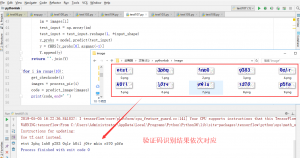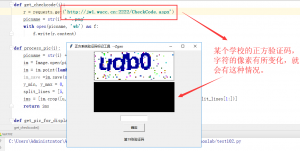im = im.point(lambda i: i == 3, mode=’1′)

#### 总结：微信赞赏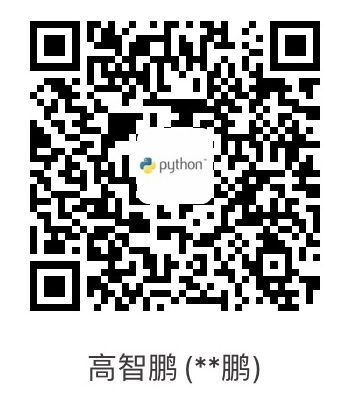支付宝赞赏